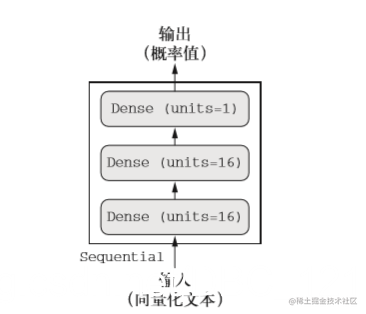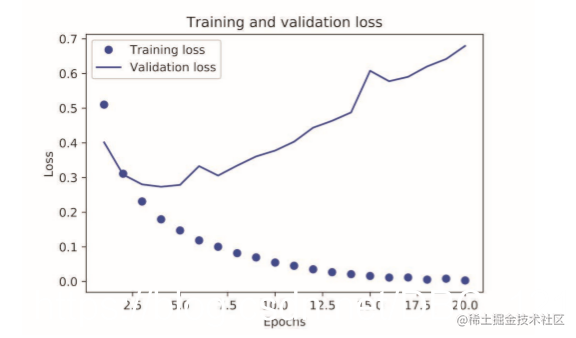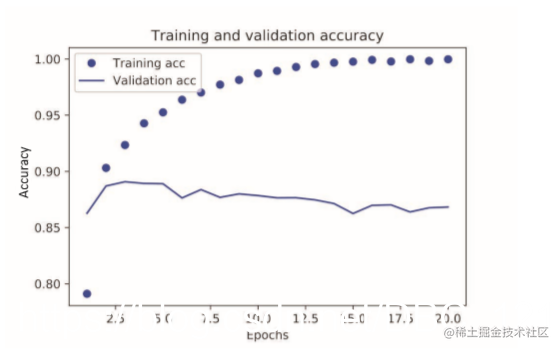# 使用TensorFlow解决电影评论分类--二分类问题

## 层

``````from tensorflow.keras import layers
layer = layers.Dense(32, input_shape(784,))

## 模型

• 双分支（two-branch）网络 +
• Inception 模块

## 损失函数与优化器

• 损失函数（目标函数）——在训练过程中需要将其最小化。它能够衡量当前任务是否已 成功完成。
• 优化器——决定如何基于损失函数对网络进行更新。它执行的是随机梯度下降（SGD） 的某个变体。

## 二分类问题

#### IMDB 数据集

``````from tensorflow.keras.datasets import imdb

(train_data, train_labels), (test_data, test_labels) = imdb.load_data(num_words=10000)

``````print( train_data)
# [1, 14, 22, 16, ... 178, 32]

print(train_labels)
# 1

# 由于限定为前 10 000 个最常见的单词，单词索引都不会超过 10 000。
print(max([max(sequence) for sequence in train_data]))
# 9999

``````word_index = imdb.get_word_index()
reverse_word_index = dict(
[(value, key) for (key, value) in word_index.items()])
decoded_review = ' '.join(
[reverse_word_index.get(i - 3, '?') for i in train_data])

print(decoded_review)

#### 准备数据

• 填充列表，使其具有相同的长度，再将列表转换成形状为 (samples, word_indices) 的整数张量，然后网络第一层使用能处理这种整数张量的层（即 Embedding 层）。
• 对列表进行 one-hot 编码，将其转换为 0 和 1 组成的向量。举个例子，序列 [3, 5] 将会被转换为10 000 维向量，只有索引为3 和 5 的元素是1，其余元素都是0。然后网络第一层可以用 Dense 层，它能够处理浮点数向量数据。 下面我们采用后一种方法将数据向量化。为了加深理解，你可以手动实现这一方法，如下所示。
``````def vectorize_sequences(sequences, dimension=10000):
results = np.zeros((len(sequences), dimension))
for i, sequence in enumerate(sequences):
results[i, sequence] = 1.
return results

x_train = vectorize_sequences(train_data)
x_test = vectorize_sequences(test_data)

y_train = np.asarray(train_labels).astype('float32')
y_test = np.asarray(test_labels).astype('float32')

#### 构建网络

``````output = relu(dot(W, input) + b)

16 个隐藏单元对应的权重矩阵 W 的形状为 (input_dimension, 16)，与 W 做点积相当于 将输入数据投影到16 维表示空间中（然后再加上偏置向量 b 并应用 relu 运算）。你可以将表 示空间的维度直观地理解为“网络学习内部表示时所拥有的自由度”。隐藏单元越多（即更高维 的表示空间），网络越能够学到更加复杂的表示，但网络的计算代价也变得更大，而且可能会导 致学到不好的模式（这种模式会提高训练数据上的性能，但不会提高测试数据上的性能）。

• 网络有多少层；
• 每层有多少个隐藏单元。

• 两个中间层，每层都有 16 个隐藏单元；
• 第三层输出一个标量，预测当前评论的情感。``````model = models.Sequential()

``````model.compile(optimizer='rmsprop',
loss='binary_crossentropy',
metrics=['accuracy'])

``````from tensorflow.keras import optimizers
model.compile(optimizer=optimizers.RMSprop(lr=0.001),
loss='binary_crossentropy',
metrics=['accuracy'])

``````from keras import losses
from keras import metrics

model.compile(optimizer=optimizers.RMSprop(lr=0.001),
loss=losses.binary_crossentropy,
metrics=[metrics.binary_accuracy])

#### 验证你的方法

``````x_val = x_train[:10000]
partial_x_train = x_train[10000:]

y_val = y_train[:10000]
partial_y_train = y_train[10000:]

``````history = model.fit(partial_x_train, partial_y_train,
epochs=20,
batch_size=512,
validation_data=(x_val, y_val))

``````history_dict = history.history
print(history_dict.keys())

``````import matplotlib.pyplot as plt

history_dict = history.history
loss_values = history_dict['loss']
val_loss_values = history_dict['val_loss']

epochs = range(1, len(loss_values) + 1)

plt.plot(epochs, loss_values, 'bo', label='Training loss')
plt.plot(epochs, val_loss_values, 'b', label='Validation loss')
plt.title('Training and validation loss')
plt.xlabel('Epochs')
plt.ylabel('Loss')
plt.legend()

plt.show()``````plt.clf()
acc = history_dict['acc']
val_acc = history_dict['val_acc']

plt.plot(epochs, acc, 'bo', label='Training acc')
plt.plot(epochs, val_acc, 'b', label='Validation acc')
plt.title('Training and validation accuracy')
plt.xlabel('Epochs')
plt.ylabel('Accuracy')
plt.legend()

plt.show()#### 使用训练好的网络在新数据上生成预测结果

``````model.predict(x_test)
#array([[ 0.98006207]
#        [ 0.99758697]
#        [ 0.99975556]
#        ...,
#        [ 0.82167041]
#        [ 0.02885115]
#        [ 0.65371346]], dtype=float32)

## 完整代码

``````from tensorflow.keras.datasets import imdb
from tensorflow.keras import layers
from tensorflow.keras import models
import matplotlib.pyplot as plt
import numpy as np

(train_data, train_labels), (test_data, test_labels) = imdb.load_data(num_words=10000)

print(max([max(sequence) for sequence in train_data]))

def vectorize_sequences(sequences, dimension=10000):
results = np.zeros((len(sequences), dimension))
for i, sequence in enumerate(sequences):
results[i, sequence] = 1.
return results

x_train = vectorize_sequences(train_data)
x_test = vectorize_sequences(test_data)

y_train = np.asarray(train_labels).astype('float32')
y_test = np.asarray(test_labels).astype('float32')

model = models.Sequential()

model.compile(optimizer='rmsprop',
loss='binary_crossentropy',
metrics=['accuracy'])

x_val = x_train[:10000]
partial_x_train = x_train[10000:]

y_val = y_train[:10000]
partial_y_train = y_train[10000:]

history = model.fit(partial_x_train, partial_y_train,
epochs=20,
batch_size=512,
validation_data=(x_val, y_val))

history_dict = history.history
loss_values = history_dict['loss']
val_loss_value = history_dict['val_loss']

epochs = range(1, len(loss_values) + 1)

plt.plot(epochs, loss_values, 'bo', label='训练损失')
plt.plot(epochs, val_loss_value, 'b', label='验证损失')
plt.title('训练和验证损失')
plt.xlabel('Epochs')
plt.ylabel('Loss')
plt.legend

plt.show()